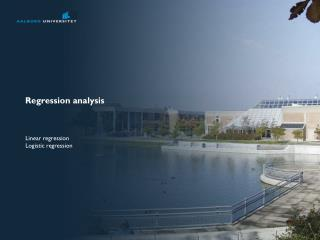# Regression analysis - PowerPoint PPT PresentationDownload PresentationRegression analysis

Download Presentation## Regression analysis

- - - - - - - - - - - - - - - - - - - - - - - - - - - E N D - - - - - - - - - - - - - - - - - - - - - - - - - - -
##### Presentation Transcript

1. Regression analysis Linear regression Logistic regression

2. Relationship and association

3. Straight line

4. Best straight line?

5. Best straight line! Least square estimation

6. Simple linear regression Is the association linear?

7. Simple linear regression Is the association linear? Describe the association: what is b0 and b1 BMI = -12.6kg/m2+0.35kg/m3*Hip

8. Simple linear regression Is the association linear? Describe the association Is the slopesignificantlydifferent from 0? Help SPSS!!!

9. Simple linear regression Is the association linear? Describe the association Is the slopesignificantlydifferent from 0? Howgood is the fit? How far are the data points fom the line onavarage?

10. The CorrelationCoefficient, r R = 0.7 R = 0 R = -0.5 R = 1

11. r2 – Goodness of fitHowmuch of the variation canbeexplained by the model? R2 = 0.5 R2 = 0 R2 = 0.2 R2 = 1

12. Multiple linear regression Couldwaistmeasuredescirbesome of the variation in BMI? BMI =1.3 kg/m2 + 0.42 kg/m3 * Waist Orevenbetter:

13. Multiple linear regression If Y is linearly dependent on more than one independent variable:  is the intercept, the value of Y when X1 and X2 = 0 1 and 2are termed partial regression coefficients 1 expresses the change of Y for one unit of X when 2 is kept constant

14. Multiple linear regression – residual error and estimations As the collected data is not expected to fall in a plane an error term must be added The error term sums up to be zero. Estimating the dependent factor and the population parameters:

15. Multiple linear regression – general equations In general an finite number (m) of independent variables may be used to estimate the hyperplane The number of sample points must be two more than the number of variables

17. Assumptions Dependent variable must be metric continuous Independent must be continuous or ordinal Linear relationship between dependent and all independent variables Residuals must have a constant spread. Residuals are normal distributed Independent variables are not perfectly correlated with each other

18. Multible linear regression in SPSS

19. Multible linear regression in SPSS

20. Non-parametric correlation

21. RankedCorrelation Kendall’s  Spearman’s rs Correlationbetween -1 og 1. Where -1 indicatesperfectinverssecorrelation , 0 indicatesnocorrelation, and 1 indicatesperfectcorrelation Pearson is the correlation method for normal data Remember the assumptions: Dependent variable must be metric continuous Independent must be continuous or ordinal Linear relationship between dependent and all independent variables Residuals must have a constant spread. Residuals are normal distributed

22. Kendall’s  - An example

23. Kendall’s  - An example

24. Spearman – the same example

25. Korrelation i SPSS

26. Korrelation i SPSS

27. Logistic regression

28. Logistic Regression • If the dependent variable is categorical and especially binary? • Use some interpolation method • Linear regression cannot help us.

29. The sigmodal curve

30. The sigmodal curve • The intercept basically just ‘scale’ the input variable

31. The sigmodal curve • The intercept basically just ‘scale’ the input variable • Large regression coefficient → risk factor strongly influences the probability

32. The sigmodal curve • The intercept basically just ‘scale’ the input variable • Large regression coefficient → risk factor strongly influences the probability • Positive regression coefficient→risk factor increases the probability • Logisticregessionusesmaximumlikelihoodestimation, not leastsquareestimation

33. Does previous intake of OCP influence the diagnosis? Categorical independent variable

34. Odds ratio

35. Multiple logistic regression

36. Predicting the diagnosis by logistic regression What is the probabilitythat the tumor of a 50 yearoldwomanwho has beenusing OCP and has a BMI of 26 is malignant? z = -6.974 + 0.123*50 + 0.083*26 + 0.28*1 = 1.6140 p = 1/(1+e-1.6140) = 0.8340

37. Logistic regression in SPSS

38. Logistic regression in SPSS